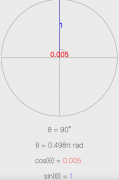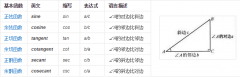•js的canvas圆形数学公式关注热度:197

•javascript常用数学公式之曲线运动关注热度:133

javascript常用数学公式之曲线运动 勾股定理 a*a + b*b =c*c 三角函数 正弦 : sin 余弦 : cos 正切 : tan 余切 : cot 正弦定理 a/sinA = b/sinB =c/sinC = 2r（r为外接圆半径） 余弦定理 cosA = b*b + c*c - a*a /..[详情]

• JavaScript中弧度和角度的转换以及相关公式关注热度:93

JavaScript中弧度和角度的转换以及相关公式 弧度 = 角度 * Math.PI / 180 角度 = 弧度 * 180 / Math.PI 在JavaScript Math 对象中: sin() 方法可返回一个数字的正弦。 tan() 方法可返回一个表示某个角的正..[详情]

•js获得圆边上点的x,y坐标关注热度:33

JS如何获得圆边上点的x,y坐标呢？ 假设已知: 半径 r ，旋转角度 angle 和 当前中心点坐标 x0,y0 如图所示： 根据三角函数： x1=x0+r*Math.cos(angle*Math.PI/180)y1=y0+r*Math.sin(angle*Math.PI/180) 假设条件..[详情]